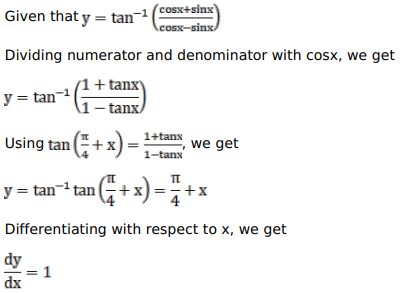# Solve this following

Question:

Mark $(\sqrt{ })$ against the correct answer in the following:

If $y=\tan ^{-1}\left\{\frac{\cos x+\sin x}{\cos x-\sin x}\right\}$ then $\frac{d y}{d x}=$ ?

A. 1

B. $-1$

C. $\frac{1}{2}$

D. $\frac{-1}{2}$

Solution: